# Rough Set Theory | Properties and Important Terms | Set – 2

PrerequisiteRough Set Theory

The main goal of the rough set analysis is the induction of approximations of concepts. Rough sets constitute a sound basis for Knowledge Discovery in Database. It offers mathematical tools to discover patterns hidden in data. It can be used for feature selection, feature extraction, data reduction, decision rule generation, and pattern extraction (templates, association rules) etc. It identifies partial or total dependencies in data, eliminates redundant data, gives approach to null values, missing data, dynamic data and others.

Four Basic Classes of Rough Sets:

There are four basic classes of Rough set thoery –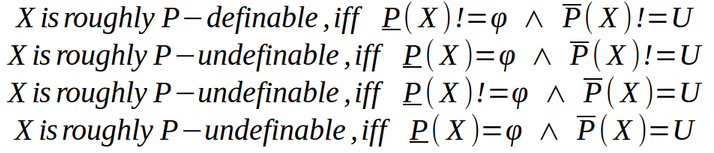Accuracy:
Accuracy of the rough set of a set X which measures how closely the rough set approximates target set X is given as –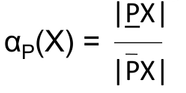where |X| denotes the cardinality of set X which is not null. Obviously, αp(X) will lie between [0, 1] –

• if αp(X)= 1, the upper and lower approximations are equal and X becomes a crisp set with respect to P.
• if αp(X)< 1, X is rough with respect to P.
• if αp(X)= 0, the the lower approximation is empty (regardless of the size of the upper approximation).

Attribute Dependency:
One of the most important aspects of database analysis is the discovery of attribute dependencies. It describes which variables are strongly related to which other variables. Set of attribute Q depends totally on a set of attributes P, denoted if all values of attributes from Q are uniquely determined by values of attributes from P. In rough set theory, the notion of dependency is defined very simply.

Let us take two disjoint sets of attributes, set P and set Q. Each attribute set induces an indiscernibility or equivalence class structure. The equivalence classes induced by P is given by [x]P and the equivalence classes induced by Q is given by [x]Q. Let, Qi is a given equivalence class from the equivalence-class structure induced by attribute set Q. The dependency of attribute set Q on attribute set P, k or γ(P, Q), is given by-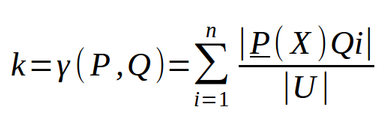Note that –

• If k or γ(P, Q)= 1, Q depends totally on P.
• If k or γ(P, Q)< 1, Q depends partially (in a degree k) on P.

Reduct:
The same or indiscernible objects may be represented several times. Some of the attributes may be superfluous or redundant. We should keep only those attributes that preserve the indiscernibility relation and consequently set approximation. There are usually several such subsets of attributes and those which are minimal are called Reduct. So a Reduct is a sufficient set of features which by itself can fully characterize the knowledge in the database. Some of the important features of Reduct are –

• Produce same equivalence class structure as that expressed by the full attribute set which can be expresses by [x]RED = [x]P.
• It is minimal.
• It is not unique.

Algorithm to Reduct Calculation –

```Input:
C, the set of all conditional features
D, the set of all decisional features

Output: R, a feature subset

1. T := { }, R : = { }
2. repeat
3. T : = R
4. ∀ x ∈ (C – R )
5. if γ RU{X} ( D ) > γT( D )
6. T : = R U {x}
7. R : = T
8. until γR( D ) = γC( D )
9. return R
```

Core:
Core is the set of attributes which is common to all reducts and denoted by CORE(P) = ∩ (RED(P)). Some of the important features of Core are –

• It consists of attributes which cannot be removed without causing collapse of the equivalence class structure.
• It may be empty.
• It is the set of necessary attributes. If we remove the core attributes from the information table, it will result in Data inconsistency.

### An Example of Reducts & Core –

Information table – In Rough Set, data model information is stored in a table. Each row represents a fact or an object. In Rough Set terminology a data table is called an Information System. Thus, the information table represents input data, gathered from any domain. Let us take an Information table as –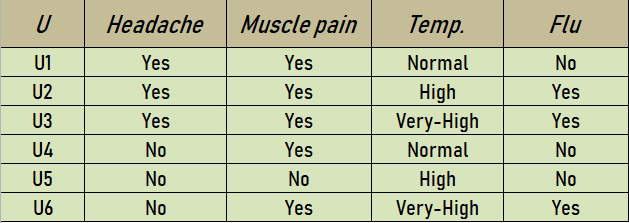Reduct Calculation:

The set {Muscle-pain, Temp.} is a reduct of the original set of attributes {Headache, Muscle_ pain, Temp.}. So, Reduct1 = {Muscle-pain, Temp.}. A new information table based on this Reduct1 is represented as –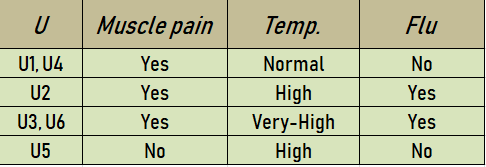The set {Headache, Temp.} is a reduct of the original set of attributes {Headache, Muscle_ pain, Temp.}. So, Reduct2 = {Headache, Temp.}. A new information table based on this Reduct2 is represented as –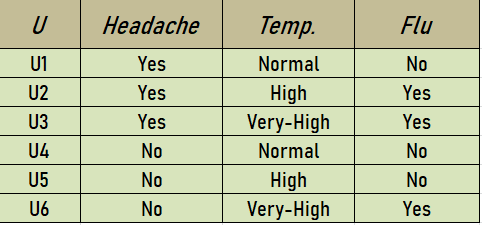So the core will be intersection of all the reducts. CORE = {Headache, Temp}∩ {MusclePain, Temp} = {Temp}

My Personal Notes arrow_drop_upCheck out this Author's contributed articles.

If you like GeeksforGeeks and would like to contribute, you can also write an article using contribute.geeksforgeeks.org or mail your article to contribute@geeksforgeeks.org. See your article appearing on the GeeksforGeeks main page and help other Geeks.

Please Improve this article if you find anything incorrect by clicking on the "Improve Article" button below.